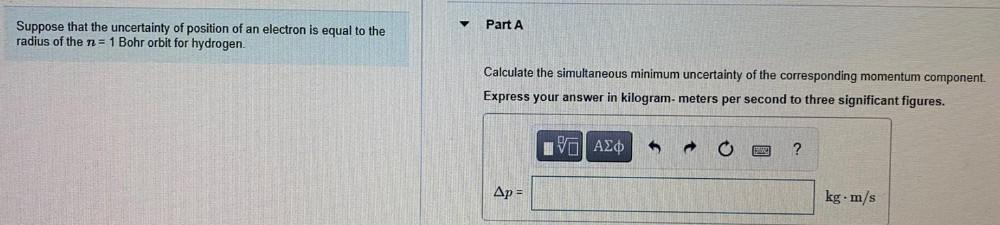Question:

# Part A Suppose that the uncertainty of position of an electron is equal to the radius of the n= 1 Bohr orbit for hydrogen. CalcuPart A Suppose that the uncertainty of position of an electron is equal to the radius of the n= 1 Bohr orbit for hydrogen. Calculate the simultaneous minimum uncertainty of the corresponding momentum component. Express your answer in kilogram-meters per second to three significant figures. IVO AO ? Ap = kg. m/s